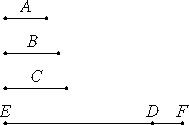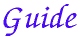Proposition 20

Prime numbers are more than any assigned multitude of prime numbers.

Let A, B, and C be the assigned prime numbers.

I say that there are more prime numbers than A, B, and C.

Take the least number DE measured by A, B, and C. Add the unit DF to DE.

Then EF is either prime or not.

First, let it be prime. Then the prime numbers A, B, C, and EF have been found which are more than A, B, and C.VII.31

Next, let EF not be prime. Therefore it is measured by some prime number. Let it be measured by the prime number G.

I say that G is not the same with any of the numbers A, B, and C.

If possible, let it be so. Now A, B, and C measure DE, therefore G also measures DE. But it also measures EF. Therefore G, being a number, measures the remainder, the unit DF, which is absurd.

Therefore G is not the same with any one of the numbers A, B, and C. And by hypothesis it is prime. Therefore the prime numbers A, B, C, and G have been found which are more than the assigned multitude of A, B, and C.

Therefore, prime numbers are more than any assigned multitude of prime numbers.

Q.E.D.This proposition states that there are more than any finite number of prime numbers, that is to say, there are infinitely many primes.

Outline of the proof

Suppose that there are n primes, a1, a2, ..., an. Euclid, as usual, takes an specific small number, n = 3, of primes to illustrate the general case. Let m be the least common multiple of all of them. (This least common multiple was also considered in proposition IX.14. It wasn’t noted in the proof of that proposition that the least common multiple of primes is their product, and it isn't noted in this proof, either.)

Consider the number m + 1. If it’s prime, then there are at least n + 1 primes.

So suppose m + 1 is not prime. Then according to VII.31, some prime g divides it. But g cannot be any of the primes a1, a2, ..., an, since they all divide m and do not divide m + 1. Therefore, there are at least n + 1 primes. Q.E.D.

This proposition is not used in the rest of the Elements.